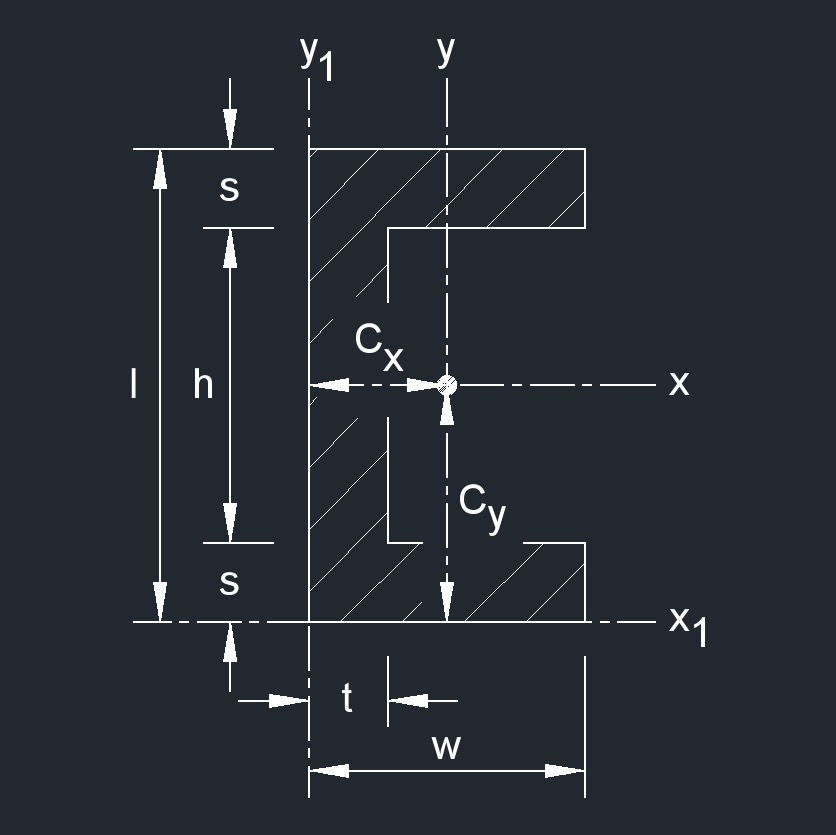# Square Channel

Written by Jerry Ratzlaff on . Posted in Plane Geometry

•Three rectangles that intersect at 90° angles at each end of one rectangle.
• A square channel is a structural shape used in construction.

### area of a Square Channel formula

$$\large{ A = w\;l - h \; \left( w - t \right) }$$

Where:

$$\large{ A }$$ = area

$$\large{ h }$$ = height

$$\large{ l }$$ = height

$$\large{ t }$$ = thickness

$$\large{ w }$$ = width

### Distance from Centroid of a Square Channel formula

$$\large{ C_x = \frac{ 2\;w^2 \;s \;+\; h\;t^2 }{ 2\;w\;l \;-\; 2\;h \; \left( w \;-\; t \right) } }$$

$$\large{ C_y = \frac{ l }{ 2} }$$

Where:

$$\large{ C }$$ = distance from centroid

$$\large{ h }$$ = height

$$\large{ l }$$ = height

$$\large{ s }$$ = thickness

$$\large{ t }$$ = thickness

$$\large{ w }$$ = width

### Elastic Section Modulus of a Square Channel formula

$$\large{ S_{x} = \frac{ I_{x} }{ C_{y} } }$$

$$\large{ S_{y} = \frac{ I_{y} }{ C_{x} } }$$

Where:

$$\large{ S }$$ = elastic section modulus

$$\large{ C }$$ = distance from centroid

$$\large{ I }$$ = moment of inertia

### Perimeter of a Square Channel formula

$$\large{ P = 2 \; \left( 2\;w + l \; \right) - 2\;t }$$

Where:

$$\large{ P }$$ = perimeter

$$\large{ l }$$ = height

$$\large{ s }$$ = thickness

$$\large{ t }$$ = thickness

$$\large{ w }$$ = width

### Polar Moment of Inertia of a Square Channel formula

$$\large{ J_{z} = I_{x} + I_{y} }$$

$$\large{ J_{z1} = I_{x1} + I_{y1} }$$

Where:

$$\large{ J }$$ = torsional constant

$$\large{ I }$$ = moment of inertia

### Radius of Gyration of a Square Channel formula

$$\large{ k_{x} = \sqrt { \frac{ w\;l^3 \;-\; h^3 \; \left( w \;-\; t \right) }{ 12 \; \left[ w\;l \;-\; h \; \left( w \;-\; t \right) \right] } } }$$

$$\large{ k_{y} = \sqrt { \frac{ I_{y} }{ A } } }$$

$$\large{ k_{z} = \sqrt { k_{x}{^2} + k_{y}{^2} } }$$

$$\large{ k_{x1} = \sqrt { \frac{ I_{x1} }{ A } } }$$

$$\large{ k_{y1} = \sqrt { \frac{ I_{y1} }{ A } } }$$

$$\large{ k_{z} = \sqrt { k_{x1}{^2} + k_{y1}{^2} } }$$

Where:

$$\large{ k }$$ = radius of gyration

$$\large{ A }$$ = area

$$\large{ I }$$ = moment of inertia

$$\large{ h }$$ = height

$$\large{ l }$$ = height

$$\large{ t }$$ = thickness

$$\large{ w }$$ = width

### Second Moment of Area of a Square Channel formula

$$\large{ I_{x} = \frac{ w\;l^3 \;-\; h^3 \; \left( w \;-\; t \right) }{ 12 } }$$

$$\large{ I_{y} = \frac{ 2\;s\;w^3 \;+\; h\;t^3 }{ 3 } - A\;C_{x}{^2} }$$

$$\large{ I_{x1} = I_{x} + A\;C_{y}{^2} }$$

$$\large{ I_{y1} = I_{y} + A\;C_{x}{^2} }$$

Where:

$$\large{ I }$$ = moment of inertia

$$\large{ A }$$ = area

$$\large{ C }$$ = distance from centroid

$$\large{ h }$$ = height

$$\large{ l }$$ = height

$$\large{ s }$$ = thickness

$$\large{ t }$$ = thickness

$$\large{ w }$$ = width

### Torsional Constant of a Square Channel formula

$$\large{ J = \frac{ 2 \; \left( w \;-\; \frac{t}{2} \right) \; s^3 \; \left( l \;-\; s \right) \; t^3 }{ 3 } }$$

Where:

$$\large{ J }$$ = torsional constant

$$\large{ l }$$ = height

$$\large{ s }$$ = thickness

$$\large{ t }$$ = thickness

$$\large{ w }$$ = width